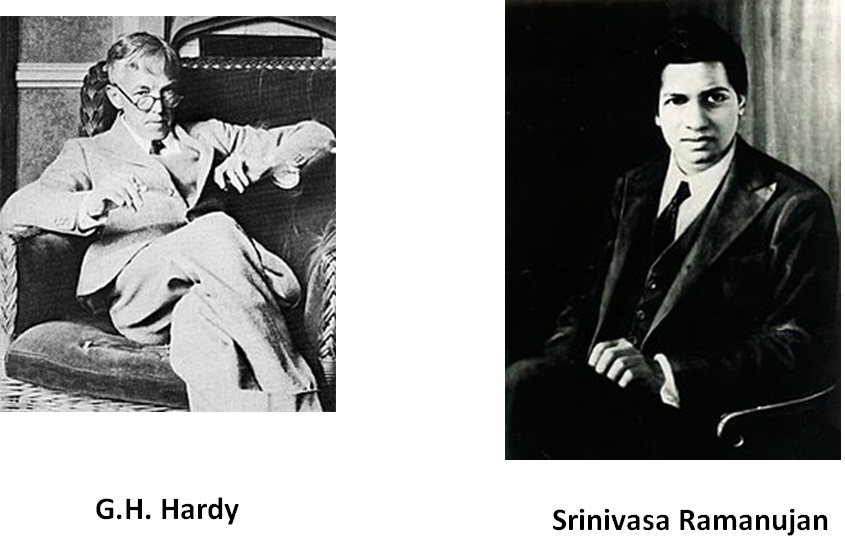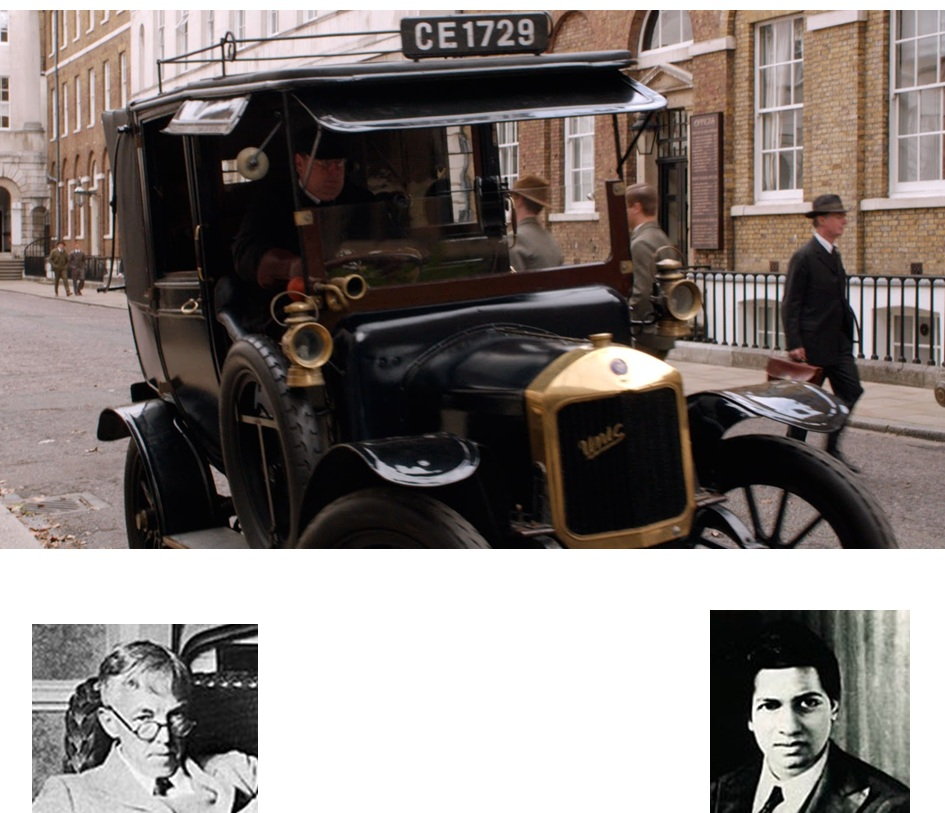Cubes

Chapter 6 Class 8 Cubes and Cube Roots
Concept wiseOnce Ramanujan, who was in London, was sick.

Hardy came to visit him in a Taxi.

This was the conversationThat’s a very dull number

From then on,

number 1729 is known as Hardy-Ramanujan Numbers

1729 can be expressed as

1729 = 1 + 1728 = 1 3 + 12 3

Or

1729 = 729 + 1000 = 9 3 + 10 3

Thus,

1729 is the smallest number that can be represented as sum of two cubes in two different ways

Learn in your speed, with individual attention - Teachoo Maths 1-on-1 Class